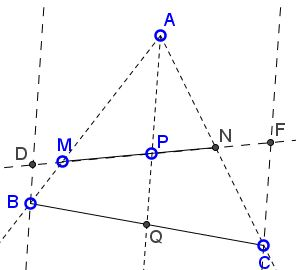# A Property of a Median

### Problem

In $\Delta ABC,\;$ $Q\;$ is the midpoint of $BC;\;$ $P\;$ a point on $AQ.\;$ A line through $P\;$ meets $AB\;$ in $M,\;$ $AC\;$ in $N.$Prove that $\displaystyle\frac{BM}{MA}+\frac{CN}{NA}\;$ is independent of the line $MN.$

Note: The lengths of the segments involved are thought to be defined algebraically. For example, for $M\in AB,\;$ $BM\;$ and $MA\;$ are of the same sign; otherwise, their signs are different.

### Proof 1

Through $B\;$ and $C\;$ draw lines parallel to $AQ.\;$ Let them cross $MN\;$ in $Q\;$ and $F,\;$ respectively.$\displaystyle\frac{BM}{AM} + \frac{CN}{AN} = \frac{BQ}{AP} + \frac{CF}{AP} = \frac{BQ+CF}{AP}\;$ and $\displaystyle PQ = \frac{BQ + CF}{2}.\;$ Hence, $\displaystyle\frac{BM}{AM} + \frac{CN}{AN} = 2\frac{PQ}{AP}.\;$ Therefore, $\displaystyle\frac{BM}{AM} + \frac{CN}{AN}\;$ is constant . Note that for the case $P=G,\;$ the centroid of $\Delta ABC,\;$ the constant is $1.$

### Proof 2

Place weight $y\;$ in $A,\;$ $x\;$ in $B\;$ and $C.\;$ Then $\displaystyle\frac{BM}{MA}=\frac{CN}{NA}=\frac{x}{y};\;$ the sum is $\displaystyle\frac{2x}{y}.\;$ From $y\cdot AP=2x\cdot PQ\;$ we get that $\displaystyle\frac{2x}{y}=\frac{AP}{PQ}=\frac{BM}{MA}+\frac{CN}{NA}.$

### Acknowledgment

The problem (with $M\;$ and $N\;$ restricted to the sides of the triangle) and the solution have been posted in a comment at one of the pages at this site as a generalization of the statement on that page. However, due to a bug in the software supporting comments, the specific page where the comment has been posted was not reported. The post with the proof (Proof 1) was by G. S. Ranaganth. Proof 2 is by Alexander Price.

### Generalization

Leo Giugiuc credits the great Romanian mathematician D. Mihaileanu with the following statement:

Let $ABC\;$ be a triangle and $P\;$ a fixed point inside it. Let $Q\;$ be the intersection of $AP\;$ with $BC.\;$ Assume $\displaystyle\frac{BQ}{QC}=\frac{z}{y}.\;$ An arbtrary line through $P\;$ cuts the sides $AB\;$ and $AC\;$ at $M\;$ and $N,\;$ respectively. Then $\displaystyle y\cdot\frac{BM}{MA}+z\cdot\frac{CN}{NA}\;$ is constant.

I can only add that allowing for algebraic segment lengths lets $P\;$ lie outside $\Delta ABC.$